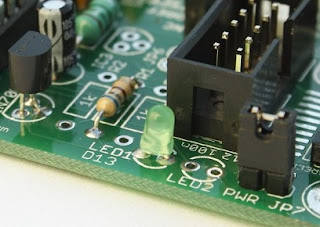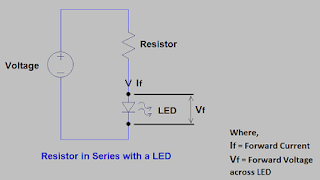# Resistor for LED Calculator

## Resistor for LED Calculator

 Input Voltage (V) LED Forward Current (mA) LED Forward Voltage Drop (V) Current Limiting Resistor (Ω)

In almost all applications where LED’s are used, a resistor in series with LED is connected. Selection of resistor for LED is not a difficult task only step wise process has to follow which is given in this article.

Also, Resistor for LED Calculator has been provided which will help you to calculate the LED resistor in easy way. Also, you can cross check your calculated LED resistor value is matching with the value generated by LED resistor calculator. This calculator for LED resistor is very useful and easy to use.Resistor for LED calculator

### Click below button to get Resistor for LED Calculator or LED Resistor Calculator in Excel fileResistor for LED calculator
Now let discuss the steps to calculate Resistor for LED and same steps has been used to develop Resistor for LED Calculator;

### Use of Resistor for LED’s

LEDs are more common for almost many of applications as an indicator or light. This is because it has good power efficiency. Also, it has good life span as compare to incandescent or fluorescent lamps.

Full form of LED is Light Emitting Diode. It’s a type of diode. Like diode it also has polarity i.e. anode and cathode. With suitable application of power across it i.e. the power matching to LED specifications, LED works and produce light.

We have to follow the polarity requirement of the LED, same like diode otherwise it will fail. Because LED allows low value of reverse polarity voltage approximately 5 volts.

Know you know that LED is a diode so, the current across the LED should not cross the limit otherwise it will cause failure to the LED.

This is the reason why resistor in the series with the LED is used. In simple words the LED resistor controls the current across the LED.Resistor with LED
Now let see the necessities or requirements for the current control resistor that must be used with a LED;

We know that LED’s are available in different colours. Each colour LED is made by different materials and these material have different voltage requirements. So, each colour LED has different electrical requirements and hence different specifications.

In LED specifications we refer a term, “Forward Voltage (Vf)”. This is the voltage which makes LED to work.

Below are forward voltage requirements of some common LED’s;

• Red = approximately 1.7 volts
• Green = approximately 2.2 volts
• Orange = approximately 2.0 volts
• Yellow = approximately 2.1 volts
• White = approximately 3.2 volts
• Blue = approximately 3.2 volts
Now we can clearly see the variation in the forward voltage. By knowing the forward voltage, we have to pass current in proper amount across the LED. So, resistor in series with LED is used, where resistor controls the current according to forward voltage.

### Correct resistor for LED

Without using a resistor, if we apply direct DC voltage to a LED, it will work.

But if the applied voltage and current is not matching to the requirement of LED, the LED will glow dim or bright or may be it get heated and fails.

In simple words, less current across LED, the LED will glow dim. More current across LED, the LED will glow bright.

If current crosses the electrical requirement of LED, the LED will fail.

So, a resistor we have to connect in series with the LED whose current we want to control.

### Calculation for selection of LED resistorResistor in series with LED
The resistor selection depends on Ohm’s law i.e. R = V/I.

V is the applied DC voltage. This can be calculated as; V = (applied Vdc) – Vf.

I is the amount of current which should flow across the LED to make it ON.

Applied DC voltage should me more than forward voltage (Vf) of LED. i.e. if you apply 12Vdc and LED has Vf of 3.2V so, a drop of 3.2V is easily possible across the LED and it works. But less than 3.2 will never make LED to glow.

The typical forward voltage and forward current requirement of LED you can easily find in LED specification sheet.

Now our formula becomes;

R = (Vdc – Vf) / If

Now let try with an example;

Vdc = 12V

Vf = 3.2V

If = 0.010A

Then,

R = (12 – 3.2) / 0.010

R = 880 ohm. Select standard resistor value; R = 910 ohm.

Now calculate the power rating of resistor.

P = V x I

P = (12 – 3.2) x 0.010

P = 0.088W

We can select 0.25W for this application as it is more than the calculated one. If we use the wattage rating less then calculated value, the resistor become hot.

While creating "Resistor for LED Calculator" we followed same formulas.

### Many LED’s in a circuit

Consider a case, there’s a requirement of many LED’s in an application.

We can use multiple LED’s of same colour or different colour in parallel connection.

By this same voltage from voltage source will be available at each LED string, but the current requirement will increase according to number of LED’s.

As current increase the wattage requirement of each resistor will also increase.

Let understand this by an example;

Vdc = 12V

Vf = 3.2V

Number of LED strings in parallel = 5

Single LED current requirement = 0.010A

Total current requirement, I =5 x 0.010 = 0.050A

Using the formula;

R = (12 – 3.2)/0.050

R = 176 ohm. Select standard resistor value; R = 180 ohm.

Wattage we can calculate;

P = V x I = (12 – 3.2) x 0.050 = 0.44

We can select 0.25W for this application as it is more than the calculated value.

For different colour LED’s we have to calculate current requirement of each string and add all current.

In this case also voltage will be same and we have to subtract forward voltage from supply voltage.

Calculation of power rating of resistor value will remain same like previous performed calculations. As mentioned before you have to select high wattage resistor as compare to calculated one.

In "Resistor for LED Calculator" we had already included the calculator for both resistor for LED in series and resistor for LED in parallel.